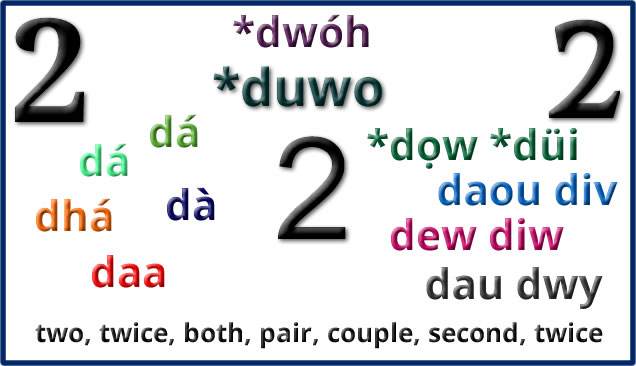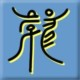# A Pair of Twos

Words for two and related things in Celtic languages:Proto-Celtic *duwo = two *dwau = two (masculine) *dwī = two (feminine) dá [daː] = two dechenc = two people déide = two things dá = two, twice dechenc = a pair of persons déide = double, consisting of two, two things, a pair dhá [ɣɑː/ɣaː] = two dá [d̪ˠɑː/d̪ˠaː] = two (used after an (the), aon (any) and céad (first)) dó = two (used when counting, e.g. a dó) dháréag = twelve daichead = forty déidhe = two persons or things, two, pair dís = two, pair, couple dà [daː] = two dà reug = twelve dà fhichead = forty dà-fhillte = double, twice over, two-way, twofold dà-chànanach = bilingual dà-chomhaireach = bidirectional dà-bheathach = amphibian dà-bheulach = duplicitous, two-faced daa [d̪eː/d̪ɛː] = two, both daa cheayrt = doubly, twice, twice over, double daa-hengagh, daa-hengoil = bilingual daa hroagh = two-way daa laare = double-decker daa lout = two-storey daa yeig = twelve daeed = forty *dọw = two (masculine) *düi = two (feminine) dau, dou, deu, dev = two (masculine) dwy = two (feminine) dau dafodioc = double-tongued, two-tongued, bilingual deu dyblyc, deudeblyg, deudyblyc, deudyblic = twofold, double, duplicate deu eiryawc = deceitful, double-tongued doudec, deudec, deudeg = twelve, dozen deuckeyn, deugeint, deu vgein, deugein = forty duyweith, dwyweith, dwyweyth = twice, doubly dau [daɨ̯/dai̯] = two (masculine), both, pair, couple, second, twice dwy [duːɨ̯/dʊi̯] = two (feminine) daudafodiog = double-tongued, two-tongued, bilingual dauddeall = equivocal, ambiguous dauddyblaf, dauddyblu = to (re)double dauddyblyg, deuddyblyg = twofold, double, duplicate daueiriog, deueiriog = deceitful, double-tongued, prevaricating, false, equivocal deuddeg = twelve, dozen deugain = forty dwywaith = twice, doubly dew = two (masculine), pair, couple dui, dyw = two (feminine) dewdhec = twelve dewugens = two score, forty deweth, dewyth = twice dew = two (masculine) diw = two (feminine) dewdhek = dozen, twelve dew ugens = forty dewblek = double, twofold dewbries = married couple dewdhen = couple, pair dewek = binary diwweyth = twice dou = two daou, dou, deü = two div = two (feminine) doudec, douzec, daoudec, daouzek = twelve dou vguent, daouuguent, daou-ugent = forty daouad = duo daudroadecq, daoudriadeg, daou-droadek = biped daougementiñ = to double daou [dɔw] = two (masculine), double, couple div [diw] = two (feminine) daouzek = twelve daou-ugent = forty daouad = binomial, duo daouhanteriñ = to cut in two di(v) wezh = twice

Etymology: possibly from Proto-Indo-European *dwóh₁ (two) [source]. English words from the same roots include binary, double, duo, duplex, twig, twin and two [source].

## 3 thoughts on “A Pair of Twos”

1.Robert says:

Simon, I see that in your graphic that displays various forms of Two, you show “twice” TWICE.

I see a new song deriving from this:

♪ Oh twice I strode down the lane a-twice
♪ And twice I met upon your eyes a-twice
♪ And twice I asked of you a kiss a-twice
♪ And one you did, my dear
♪ And glee of glees
♪ Oh once you did, my dear

All you need now is some music
And all you need now is music

Cheers,
Robert

2.Simon says:

Twice twice – I’ll pretend that was deliberate.

The song has potential. You could also add a thrice or three.

3.Robert says:

Oh what a dilemma of a quandry! Whatever shall we say should we grasp (at straws?) beyond our reach and seek a forth refrain?

Force? Fourthse? Fourth’s? Four-sooth? Foursome? Force-some?